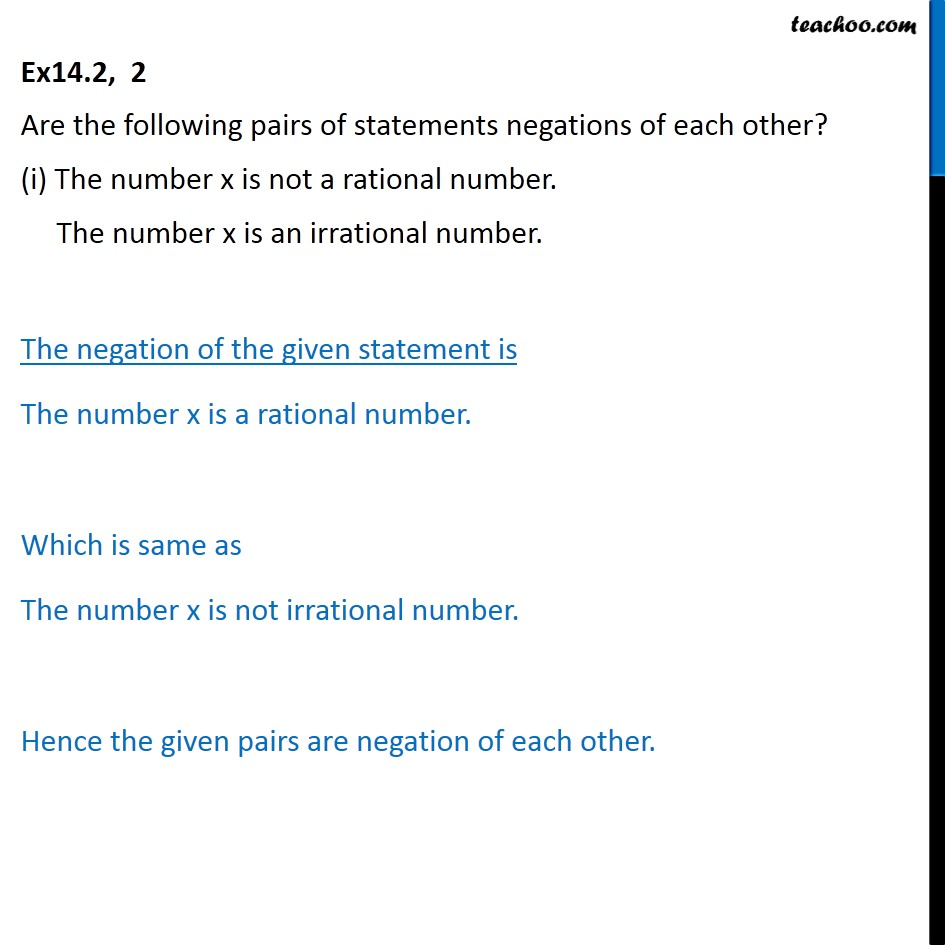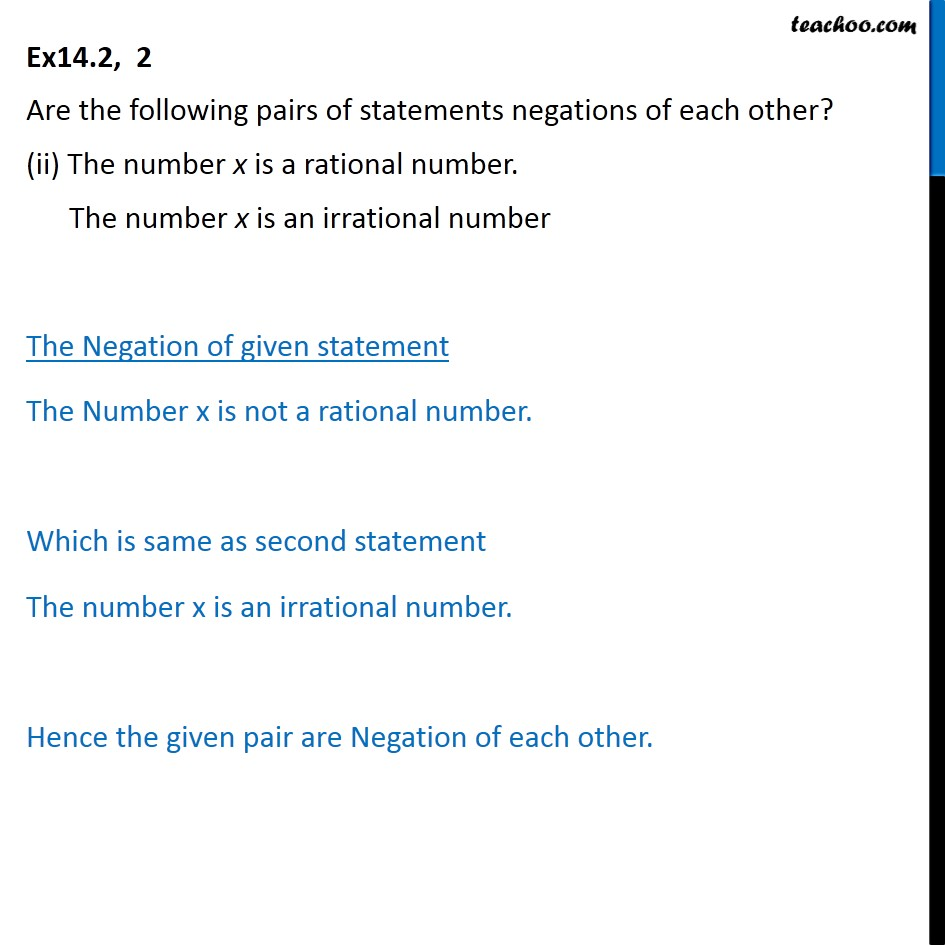Subscribe to our Youtube Channel - https://you.tube/teachoo

1. Chapter 14 Class 11 Mathematical Reasoning
2. Serial order wise

Transcript

Ex14.2, 2 Are the following pairs of statements negations of each other? (i) The number x is not a rational number. The number x is an irrational number. The negation of the given statement is The number x is a rational number. Which is same as The number x is not irrational number. Hence the given pairs are negation of each other. Ex14.2, 2 Are the following pairs of statements negations of each other? (ii) The number x is a rational number. The number x is an irrational number The Negation of given statement The Number x is not a rational number. Which is same as second statement The number x is an irrational number. Hence the given pair are Negation of each other.